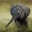Related Tags

c++
coding challenge

# How to group even and odd nodes together in a linked listChayshta Singh

In this article, we are going to learn how to rearrange the nodes in a singly linked list so that its odd elements come before its even elements.

### Algorithm

The basic idea is to split the list into two separate lists for even elements and odd elements, and then to append the even elements to the odd elements.

Input

As 2 is even, it is appended to the even list and current is moved to the next node.

The next node's value is 1 which is odd. Hence, it is appended to the odd list and current is moved to the next node.
The value at current is 4, which is even, so it as appended to the even list and current is moved to the next node.

The value at current node is 3 here, which is odd, so it is appended to the odd list and current is moved to the next node.

To prevent loops, the node next to node 4 is set to NULL.
The even elements are appended to odd elements.

### Complexity analysis

The time complexity of this algorithm is O(N) where N is the number of nodes in the input linked list.

### Code example

Node.h
#include <iostream>
#include "Node.h"
using namespace std;

//Rearrange a linked list such that all the odd elements come before the even elements
Node *prevOdd = nullptr, *prevEven = nullptr;
while(current){
// add current in odd elements list
if(current->data % 2 == 1){
if(prevOdd)
prevOdd->next = current;
else
prevOdd = current;
}
else {
if(prevEven)
prevEven->next = current;
else
prevEven = current;
}
current = current->next;
}
//prevEven->next may be nullptr or an odd node.
prevEven->next = nullptr;

//append even elements to odd elements
else
}

//arr will be used for list1 values
int arr[] = { 1,2,4,3,5,7,8,6,9};
int size = 9;

C++ Implementation

### Explanation

In main.cpp, we do the following:

• Lines 11–28: We traverse a list where current is the current node.
• Lines 13–18: We point to the last added odd node with prevOdd. Since current has an odd value, current is appended to the odd list and prevOdd is updated to point to current.
• Lines 21–25: We point to the last added even node with prevEven. Since current has an even value, current is appended to the even list and prevEven is updated to point tocurrent.
• Lines 30–31: We point to the last even node with prevEven. We also see that prevEven->next may be nullptr or an odd node. It is updated to NULL to prevent loops.
• Lines 34–37: We append even elements to odd elements where oddHead or evenHead may point to empty lists.

RELATED TAGS

c++
coding challenge

CONTRIBUTORChayshta Singh
RELATED COURSES

View all Courses

Keep Exploring

Learn in-demand tech skills in half the time